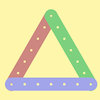#### You may also like### I'm Eight

Find a great variety of ways of asking questions which make 8.### Let's Investigate Triangles

Vincent and Tara are making triangles with the class construction set. They have a pile of strips of different lengths. How many different triangles can they make?### Noah

Noah saw 12 legs walk by into the Ark. How many creatures did he see?

# Making Longer, Making Shorter

##### Age 5 to 7Challenge Level

Thank you to everyone who submitted solutions to this problem. Some, like the Primary Two Class at Glencairn Primary, used a practical approach; the children in this class used cubes to make the different rods. The children in Primary Three had been learning their times tables, and so they used these to help them solve the problem. For example, Ewan said:

I used my $4$ times table to find the answer when we were making longer rods. e.g. $4\times2 = 8$, $4\times3=12$ and $4\times4=16$.

Sophie said:

I used my knowledge of the $2$ and $4$ times tables to find the shorter rods. Half of $4$ is $2$ and a quarter of $4$ is $1$.

Ardonis, Chioma, Phoebe and Nala, from Holy Trinity C of E Primary School submitted a lovely and clear solution. They noticed that "twice the length" meant "multiply by two", and "half the length" meant "divide by two". Here are their answers:

Ahmed's rod is four cubes long

How many cubes did he need to make a rod twice the length of that one? $4\times2=8$
How many cubes did he need to make one three times the length? $4\times3=12$
How many cubes did he need to make one four times the length? $4\times4=16$
How many cubes did he need to make a rod half the length of his first one? $4\div2=2$
How many cubes did he need to make a rod a quarter of the length of his first one? $4\div4=1$

Elyse from Putney High also submitted a correct answer, as did Reema from the British School in Dubai. Reema looked at the rods that Ahmed made, and matched them up with the descriptions:

the first picture of blocks is equal to Ahmed's
the second is half of Ahmed's blocks
the third is a quarter
the fourth is twice Ahmed's blocks
the fifth is three times Ahmed's blocks
the last one is four times Ahmed's blocks

Well done also to Padraic and Aoibheann from Cloghans Hill NS in Ireland who sent in a correct solution.

Thank you very much to those who submitted solutions. Well done!